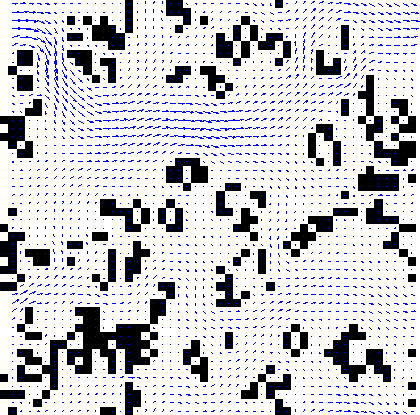If any man will draw up his case, and put his name at the foot of the first page, I will give him an immediate reply. Where he compels me to turn over the sheet, he must wait my leisure.
John Montagu, 4th Earl of Sandwich

Nick Trefethen has suggested that numerical programming can often best be accomplished through Ten Digit Algorithms, for which ten digits of accuracy should be achieved within one page of code to run in under five seconds. I strongly believe that such code can aid clarity, communication and experimentation. It is also fun to write. While software often seems to be designed with the philosophy that it’s perfected when there’s nothing left to add, TDAs are perfected when there’s nothing left to take away.

I have written a lattice Boltzmann code for fluid flow, whose implementation fits on a single page and runs in five seconds, producing useful results. To my knowledge, this is the most concise implementation of LBM, and could serve as an introduction to the method.

I chose to write it in Matlab, since its support for array operations and visualisation made the code clearer and concise.

## 2-D Model of Porous Medium Flows

``````% 2D Lattice Boltzmann (BGK) model of a fluid.
%  c4  c3   c2  D2Q9 model. At each timestep, particle densities propagate
%    \  |  /    outwards in the directions indicated in the figure. An
%  c5 -c9 - c1  equivalent 'equilibrium' density is found, and the densities
%    /  |  \    relax towards that state, in a proportion governed by omega.
%  c6  c7   c8      Iain Haslam, March 2006.
omega=1.0; density=1.0; t1=4/9; t2=1/9; t3=1/36; c_squ=1/3; nx=31; ny=31;
F=repmat(density/9,[nx ny 9]); FEQ=F; msize=nx*ny; CI=[0:msize:msize*7];
%a=repmat(-15:15,[31,1]);BOUND=(a.^2+a'.^2)<16;BOUND(1:nx,[1 ny])=1;
%BOUND=zeros(nx,ny);BOUND(1:nx,1)=1;%open channel
BOUND=rand(nx,ny)>0.7; %extremely porous random domain
ON=find(BOUND); %matrix offset of each Occupied Node
TO_REFLECT=[ON+CI(1) ON+CI(2) ON+CI(3) ON+CI(4) ...
ON+CI(5) ON+CI(6) ON+CI(7) ON+CI(8)];
REFLECTED= [ON+CI(5) ON+CI(6) ON+CI(7) ON+CI(8) ...
ON+CI(1) ON+CI(2) ON+CI(3) ON+CI(4)];
avu=1; prevavu=1; ts=0; deltaU=1e-7; numactivenodes=sum(sum(1-BOUND));
while (ts<4000 & 1e-10<abs((prevavu-avu)/avu)) | ts<100
% Propagate
F(:,:,4)=F([2:nx 1],[ny 1:ny-1],4);F(:,:,3)=F(:,[ny 1:ny-1],3);
F(:,:,2)=F([nx 1:nx-1],[ny 1:ny-1],2);F(:,:,5)=F([2:nx 1],:,5);
F(:,:,1)=F([nx 1:nx-1],:,1);F(:,:,6)=F([2:nx 1],[2:ny 1],6);
F(:,:,7)=F(:,[2:ny 1],7); F(:,:,8)=F([nx 1:nx-1],[2:ny 1],8);
BOUNCEDBACK=F(TO_REFLECT); %Densities bouncing back at next timestep
DENSITY=sum(F,3);
UX=(sum(F(:,:,[1 2 8]),3)-sum(F(:,:,[4 5 6]),3))./DENSITY;
UY=(sum(F(:,:,[2 3 4]),3)-sum(F(:,:,[6 7 8]),3))./DENSITY;
UX(1,1:ny)=UX(1,1:ny)+deltaU; %Increase inlet pressure
UX(ON)=0; UY(ON)=0; DENSITY(ON)=0;
U_SQU=UX.^2+UY.^2; U_C2=UX+UY; U_C4=-UX+UY; U_C6=-U_C2; U_C8=-U_C4;
% Calculate equilibrium distribution: stationary
FEQ(:,:,9)=t1*DENSITY.*(1-U_SQU/(2*c_squ));
% nearest-neighbours
FEQ(:,:,1)=t2*DENSITY.*(1+UX/c_squ+0.5*(UX/c_squ).^2-U_SQU/(2*c_squ));
FEQ(:,:,3)=t2*DENSITY.*(1+UY/c_squ+0.5*(UY/c_squ).^2-U_SQU/(2*c_squ));
FEQ(:,:,5)=t2*DENSITY.*(1-UX/c_squ+0.5*(UX/c_squ).^2-U_SQU/(2*c_squ));
FEQ(:,:,7)=t2*DENSITY.*(1-UY/c_squ+0.5*(UY/c_squ).^2-U_SQU/(2*c_squ));
% next-nearest neighbours
FEQ(:,:,2)=t3*DENSITY.*(1+U_C2/c_squ+0.5*(U_C2/c_squ).^2-U_SQU/(2*c_squ));
FEQ(:,:,4)=t3*DENSITY.*(1+U_C4/c_squ+0.5*(U_C4/c_squ).^2-U_SQU/(2*c_squ));
FEQ(:,:,6)=t3*DENSITY.*(1+U_C6/c_squ+0.5*(U_C6/c_squ).^2-U_SQU/(2*c_squ));
FEQ(:,:,8)=t3*DENSITY.*(1+U_C8/c_squ+0.5*(U_C8/c_squ).^2-U_SQU/(2*c_squ));
F=omega*FEQ+(1-omega)*F;
F(REFLECTED)=BOUNCEDBACK;
prevavu=avu;avu=sum(sum(UX))/numactivenodes; ts=ts+1;
end
figure;colormap(gray(2));image(2-BOUND');hold on;
quiver(2:nx,1:ny,UX(2:nx,:)',UY(2:nx,:)');
title(['Flow field after ',num2str(ts),'\deltat']);xlabel('x');ylabel('y');
Flow through random grid of cells``````Typical Flow Field Calculated from the 2-D LBM Code.

## 3-D Model of Porous Medium Flows

An analagous LBM in 3-D uses the same governing equations, but requires 19 velocities. This code won’t quite fit on a single page, but I think the similarities with the 2-D code, and deliberate repetition during calculation of the equilibrium particle densities redeem the second page. Repetition is clearer and more efficient than creating a single array of weightings and concatenating the velocity arrays in order to calculate FEQ in a single line.One stationary vector, six nearest neighbours, and twelve next-nearest in 3-D.

## Validation

The flow profile in a fully saturated channel is calculated using the LBM and compared with the analytical solution, to demonstrate that the method works as advertised.

Note that providing ten digits of accuracy when solving engineering problems, rather than the mathematics for which TDAs were proposed, is generally neither achievable nor required.